#### How to Calculate Real Interest Rate

In this lesson, you will learn how to calculate the real interest rate.

Calculate the real rate of interest when you are dealing with periodic interest capitalization. Otherwise, the actual rate and the nominal rate (which is given by the bank) are the same.

## Real Interest Rate calculations using Effect Function

Using the Effect function, you can calculate the real interest rate depending on the number of compounding periods per year.

To calculate the real interest rate, you need to know the nominal interest rate and the number of compounding periods per year. In this example, you will use one of the financial Effect function to calculate the real interest rate, the nominal rate of 5% and the quarterly capitalization.

Go to the Formulas tab. Select the Function Library section and click the Financial button.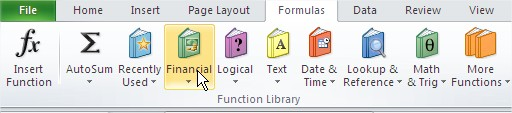Select an Effect function.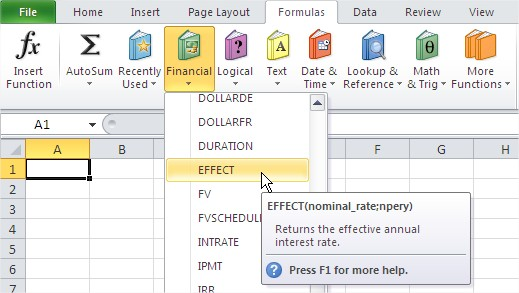A dialog box appears. Type:

• Nominal_rate – nominal interest rate, be sure to type the symbol % as a fraction or decimal, such as 7% or 0.07,
• Npery – the number of compounding periods. It will always be an integer. For example, the monthly cap – type 12, the quarterly cap – type 4.

So the calculated interest rate, simply multiplies the amount invested to calculate how much your savings will grow over the year.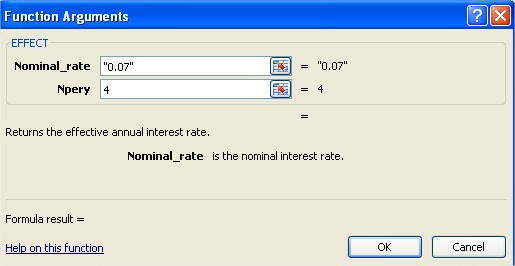The formula in this example is: =EFFECT(“0.07”,4)

The result equals 0.071859. It is the same such 7.1859%.

## Real Interest Rate calculations based on Inflation Rate

You can also calculate the Real Interest Rate the other way. Knowing the inflation rate, you are able to calculate that using the below formula:

Real Interest Rate = Nominal Interest Rate – Inflation Rate

Nominal interest rates = 5.75%

(Expected) Inflation rate = 2.25%

The task is to calculate the real interest rate based on the given data.

Real interest rate = 5.75% – 2.25% = 3.5%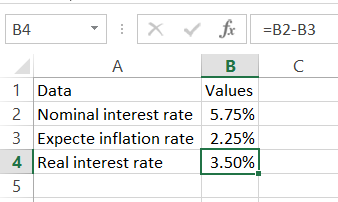The formula used is just =B2-B3.

## Example – which investment is better?

The bank offers two investments: a monthly interest rate of 15% per annum and a 15.8% annual interest rate. Find out which place is more favorable.

If you choose an investment with a one-year capitalization, after one year the deposit will increase by 15.8%.

If you choose an investment with a monthly capitalization, after one year the deposit will increase by =EFFECT(“0.15”,12). (the interest rate is 15% and the number of interest periods is equal to 12).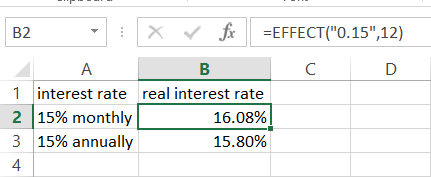The result is 16,0755%. As you can see, the monthly deposit is favorable.

The “effect” function returns the effective annual interest rate, not the monthly or quarterly interest rate. To calculate the effective interest rate for a different period, you’ll need to modify the formula accordingly.